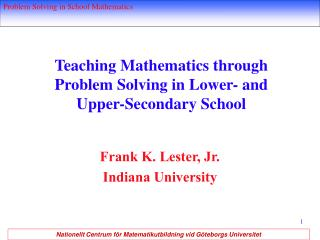DownloadDownload PresentationTeaching Mathematics through Problem Solving in Lower- and Upper-Secondary School

# Teaching Mathematics through Problem Solving in Lower- and Upper-Secondary School

Télécharger la présentation## Teaching Mathematics through Problem Solving in Lower- and Upper-Secondary School

- - - - - - - - - - - - - - - - - - - - - - - - - - - E N D - - - - - - - - - - - - - - - - - - - - - - - - - - -
##### Presentation Transcript

1. Teaching Mathematics through Problem Solving in Lower- and Upper-Secondary School Frank K. Lester, Jr. Indiana University

2. Themes for this session: The teacher’s role Developing habits of mind toward problem solving

3. The cylinders problem LAUNCH: Do cylinders with the same surface area have the same volume?

4. SUMMARIZE • Have students report about their findings. • Encourage student-to-student questions. • Look back: How is this problem related to problems we have done before? • What have we learned about the relationship between circumference and volume? • Examine the formulas for surface area and volume (Big math ideas) SA = (2π)R*H; V = πR2*H

5. Extending the Activity • Have students conjecture about what is happening to the volume as the cylinder continues to be cut, getting shorter and shorter (and wider and wider). • Some students may become interested in exploring the limit of the process of continuing to cut the cylinders in half and forming new ones. • What if the cylinders have a top and bottom?

6. Qualities of the Lesson • A question is posed about an important mathematics concept. • Students make conjectures about the problem. • Students investigate and use mathematics to make sense of the problem. • The teacher guides the investigation through questions, discussions, and instruction. • Students expect to make sense of the problem. • Students apply their understanding to another problem or task involving these concepts.

7. 12 345 678 987 654 321

8. 12 345 678 987 654 321

9. HoM 1:Mathematics is the study of patterns and structures, so always look for patterns. 11 x 11 = 121 111 x 111 = 12 321 1 111 x 1 111 = 1 234 321 11 111 x 11 111 = 123 454 321 . . . 111 111 111 x 111 111 111 = 12 345 678 987 654 321

10. Which triangles can be divided into 2 isosceles triangles?

11. Find the sum of the interior angles of the star in at least 5 different ways.

12. HoM2: Develop a willingness to receive help from others and provide help to others. • HoM3: Learn how to make reasoned (and reasonable) guesses. • HoM4: Become flexible in the use of a variety of heuristics and strategies.

13. For what values of n does the following system of equations have 0, 1, 2, 3, 4, or 5 solutions? x2 - y2 = 0 (x - n)2 + y2 = 1

14. Determine the sum of the series: 1/1•2 + 1/2•3 + 1/3•4 + 1/4•5 + 1/n•(n+1)

15. HoM 5: Draw a picture or diagram that focuses on the relevant information in the problem statement. • HoM 6: If there is an integer parameter, n, in the problem statement, calculate a few special cases for n = 1, 2, 3, 4, 5. A pattern may become evident. If so, you can then verify it by induction.

16. (A2 + 1)(B2 + 1)(C2 + 1)(D2+ 1)A•B•C•D ≥ 16 If A, B, C, and D are given positive numbers, prove or disprove that

17. If x, y, z, and w lie between 0 and 1, prove or disprove that (1 - x)(1 - y)(1 - z)(1 - w) > 1 - x - y - z - w

18. HoM 7: If there are a large number of variables in a problem, all of which play the same role, look at the analogous 1- or 2-variable problem. This may allow you to build a solution from there. • HoM 8: If a problem in its original form is too difficult, relax one of the conditions. That is, ask for a little less than the current problem does, while making sure that the problem you consider is of the same nature.

19. Suppose n distinct points are chosen on a circle. If each point is connected to each other point, what is the maximum number of regions formed in the interior of the circle?

20. HoM 9: Be skeptical of your solutions. • HoM 10: Do not do anything difficult or complicated until you have made certain that no easy solution is available.